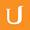## Unique Solution - Differential Equations in Action

• 0:00 - 0:03
So here is the connection with differential equations:
• 0:03 - 0:09
The exponential function is the unique solution to the following differential equation:
• 0:09 - 0:19
The derivative of Y of X, with respect to X, equals Y of X, for all X,
• 0:19 - 0:28
and the initial value, Y of zero, equals one. This is about as simple as it can get with differential equations.
• 0:28 - 0:35
We're looking for a function, Y, that is its own derivative, and starts with a value of one.
• 0:35 - 0:40
It's clear now that the exponential function is a solution to that differential equation,
• 0:40 - 0:46
but as most other differential equations that are of interest, also this differential equation is so well-behaved
• 0:46 - 0:52
that the exponential function is the unique solution of this differential equation.
Title:
Unique Solution - Differential Equations in Action
Video Language:
English
Team:Udacity
Project:
CS222 - Differential Equations
Duration:
0:53Udacity Robot edited English subtitles for Unique Solution - Differential Equations in Actionsp1 added a translation

# English subtitles

## Revisions Compare revisions

• API
Udacity Robot
• sp1